Theory and Modern Applications

# Solving certain classes of Lane-Emden type equations using the differential transformation method

## Abstract

In this paper, the differential transformation method (DTM) is applied to solve singular initial problems represented by certain classes of Lane-Emden type equations. Some new differential transformation formulas for certain exponential and logarithmic nonlinearities are derived. The approximate and exact solutions of these equations are calculated in the form of series with easily computable terms. The results obtained with the proposed methods are in good agreement with those obtained by other methods. The advantages of this technique are shown as well.

## Introduction

Singular initial value problems for Lane-Emden type equations occur in several models of mathematical physics and astrophysics  such as the theory of stellar structure, the thermal behavior of a spherical cloud of gas, isothermal gas spheres or theory of thermionic currents  which are modeled by means of the following Lane-Emden equation:

${y}^{″}\left(x\right)+\frac{\alpha }{x}{y}^{\prime }\left(x\right)+f\left(x,y\right)=g\left(x\right),\phantom{\rule{1em}{0ex}}0
(1)

with the following initial conditions:

$y\left(0\right)=a,\phantom{\rule{2em}{0ex}}{y}^{\prime }\left(0\right)=b,$
(2)

where a, b are constants, $f\left(x,y\right)$ is a continuous function and $g\left(x\right)\in C\left[0,1\right]$.

Many methods have been used to solve singular initial value problem (1), (2). For instance, Ramos  presented a series approach to the Lane-Emden equation and made comparisons with He’s homotopy perturbation method. Dehghan and Shakeri  were first to apply exponential transformation to the Lane-Emden equation in order to address the difficulty of a singular point at $x=0$ and solve the resulting nonsingular problem using the variational iteration method. Momoniat and Harley  obtained an approximate implicit solution by reducing the Lane-Emden equation to a first-order differential equation using Lie group analysis and determining a power series solution of the reduced equation. Approximate solutions of Lane-Emden type equations were presented by Shawagfeh  and Wazwaz  using the Adomian decomposition method which provides a convergent series solution. Recently Yang and Hou  proposed an approximation algorithm for the solution of a Lane-Emden type equation based on hybrid functions and the collocation method.

In this paper, the differential transformation method (DTM) is successfully applied to find an exact and approximate solution of Lane-Emden type equations with exponential and logarithmic nonlinearities. Some examples are given to demonstrate the validity and applicability of the presented method and a comparison with existing results is made.

## 1 Differential transformation method

The concept of differential transformation was first proposed by Zhou  in 1986 and it was applied to solve linear and non-linear initial value problems in electric circuit analysis. This method constructs a semi-analytical numerical technique that uses Taylor series for the solution of differential equations in the form of polynomials. It is different from the high-order Taylor series method which requires symbolic computation of the necessary derivatives of the data functions.

The method was used in a direct way without using linearization, perturbation or restrictive assumptions (see [15, 2227]). Therefore, it is not affected by computation round-off errors and one is not faced with the necessities of large computer memory and time. This method, unlike most numerical techniques, provides an exact solution. A specific advantage of this method over any purely numerical method is that it offers a smooth, functional form of the solution over a time step.

The differential transformation of the k th derivative of a function $y\left(x\right)$ is defined as follows:

$Y\left(k\right)=\frac{1}{k!}{\left[\frac{{d}^{k}y\left(x\right)}{d{x}^{k}}\right]}_{x={x}_{0}},$
(3)

where $y\left(x\right)$ is the original function and $Y\left(k\right)$ is the transformed function. Differential inverse transformation of $Y\left(k\right)$ is defined as follows:

$y\left(x\right)=\sum _{k=0}^{\mathrm{\infty }}Y\left(k\right){\left(x-{x}_{0}\right)}^{k}.$
(4)

In fact, inverse transformation (4) implies that the concept of differential transformation is derived from Taylor series expansion. Although DTM is not able to evaluate the derivatives symbolically, relative derivatives can be calculated in an iterative way which is described by the transformed equations of the original function.

From definitions (3), (4), we can derive the following:

Theorem 1 Assume that $F\left(k\right)$, $G\left(k\right)$, $H\left(k\right)$ and ${U}_{i}\left(k\right)$, $i=1,\dots ,n$, are the differential transformations of the functions $f\left(x\right)$, $g\left(x\right)$, $h\left(x\right)$ and ${u}_{i}\left(x\right)$, $i=1,\dots ,n$, respectively, thenThe proof of Theorem 1 is available in .

## 2 Numerical applications

In this section, we will investigate Lane-Emden type equations with exponential and logarithmic nonlinearities which occur in the stellar structure theory (see [6, 11, 2830]).

Theorem 2 If $f\left(y\left(x\right)\right)={e}^{ay\left(x\right)}$, ${x}_{0}=0$, $a\in \mathbb{R}$ and $F\left(k\right)$ is the differential transformation of the function $f\left(y\left(x\right)\right)$, then

$F\left(k\right)=\left\{\begin{array}{cc}{e}^{aY\left(0\right)},\hfill & k=0,\hfill \\ \frac{a}{k}{\sum }_{i=1}^{k}iY\left(i\right)F\left(k-i\right),\hfill & k\ge 1,\hfill \end{array}$
(5)

where

$Y\left(i\right)=\frac{1}{i!}{\left[\frac{{d}^{i}y\left(x\right)}{d{x}^{i}}\right]}_{x=0},\phantom{\rule{1em}{0ex}}i=1,\dots ,k.$

Proof From the definition of the transformation,

$F\left(0\right)={e}^{ay\left(x\right)}{|}_{x=0}={e}^{ay\left(0\right)}={e}^{aY\left(0\right)}.$

Put

$f\left(y\left(x\right)\right)={e}^{ay\left(x\right)}=\sum _{k=0}^{\mathrm{\infty }}F\left(k\right){x}^{k},\phantom{\rule{1em}{0ex}}y\left(x\right)=\sum _{k=0}^{\mathrm{\infty }}Y\left(k\right){x}^{k}.$
(6)

By differentiation $f\left(y\left(x\right)\right)$ with respect to x, we get

${\left(f\left(y\left(x\right)\right)\right)}^{\prime }=a{y}^{\prime }\left(x\right)f\left(y\left(x\right)\right).$
(7)

From (6) and Theorem 1, we obtain

$\sum _{k=0}^{\mathrm{\infty }}\left(k+1\right)F\left(k+1\right){x}^{k}=a\left(\sum _{k=0}^{\mathrm{\infty }}\left(k+1\right)Y\left(k+1\right){x}^{k}\right)\left(\sum _{k=0}^{\mathrm{\infty }}F\left(k\right){x}^{k}\right).$

Comparing the terms with the same power of ${x}^{k}$, we have

$\left(k+1\right)F\left(k+1\right)=a\sum _{i=0}^{k}\left(i+1\right)Y\left(i+1\right)F\left(k-i\right).$

From this, we get

$F\left(k+1\right)=\frac{a}{k+1}\sum _{i=0}^{k}\left(i+1\right)Y\left(i+1\right)F\left(k-i\right),\phantom{\rule{1em}{0ex}}k\ge 0.$
(8)

Replacing $k+1$ by k and $i+1$ by i, it follows

$F\left(k\right)=\frac{a}{k}\sum _{i=1}^{k}iY\left(i\right)F\left(k-i\right),\phantom{\rule{1em}{0ex}}k\ge 1.$

The proof is complete. □

Example 1 Consider the isothermal gas spheres equation in the case that the temperature remains constant (see [6, 12, 15, 31, 32]) which is described by the Lane-Emden type equation

${y}^{″}+\frac{2}{x}{y}^{\prime }+{e}^{y}=0$
(9)

with initial conditions $y\left(0\right)={y}^{\prime }\left(0\right)=0$.

From initial conditions and Theorem 1, we get

$Y\left(0\right)=0,\phantom{\rule{2em}{0ex}}Y\left(1\right)=0,\phantom{\rule{2em}{0ex}}F\left(0\right)=1,\phantom{\rule{2em}{0ex}}F\left(1\right)=0.$

Multiplying equation (9) by x and using Theorem 1, we obtain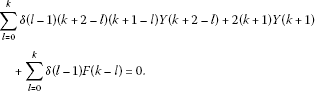(10)

From here, we obtain the recurrence relation

$Y\left(k+1\right)=-\frac{1}{\left(k+1\right)\left(k+2\right)}F\left(k-1\right),\phantom{\rule{1em}{0ex}}k\ge 1.$
(11)

Replacing $k-1$ by k, we get

$Y\left(k+2\right)=-\frac{1}{\left(k+2\right)\left(k+3\right)}F\left(k\right),\phantom{\rule{1em}{0ex}}k\ge 0.$
(12)

Using (5), we can write recurrence relation (12) in the form

$Y\left(k+2\right)=-\frac{1}{k\left(k+2\right)\left(k+3\right)}\sum _{i=1}^{k}iY\left(i\right)F\left(k-i\right),\phantom{\rule{1em}{0ex}}k\ge 0.$
(13)

Since

$F\left(k\right)=-\left(k+2\right)\left(k+3\right)Y\left(k+2\right)$

then we can write relation (13) in the form

$Y\left(k+2\right)=\frac{1}{k\left(k+2\right)\left(k+3\right)}\sum _{i=1}^{k}i\left(k-i+2\right)\left(k-i+3\right)Y\left(i\right)Y\left(k-i+2\right),\phantom{\rule{1em}{0ex}}k\ge 1.$
(14)

It is obvious that

$Y\left(2\right)=-\frac{1}{6}F\left(0\right)=-\frac{1}{6}.$

Then recurrence relation (14) gives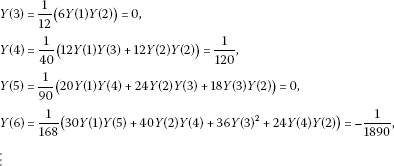Using the inverse transformation rule, we obtain an approximate solution of equation (6) in the form

$y\left(x\right)=\sum _{k=0}^{\mathrm{\infty }}Y\left(k\right){x}^{k}=-\frac{1}{6}{x}^{2}+\frac{1}{120}{x}^{4}-\frac{1}{1890}{x}^{6}+\cdots .$

Batiha , Gupta , Rafig et al. , Yildirim et al. , Parand et al.  obtained the same result by the variation iteration method, the homotopy perturbation method and the Hermite functions collocation method but using symbolic calculations as integral iterative functionals and solving differential equations of the second order.

Example 2 Now, we consider a more general type of equation (9)

${y}^{″}+\frac{2}{x}{y}^{\prime }+4\left(2{e}^{y}+{e}^{\frac{y}{2}}\right)=0$
(15)

with initial conditions $y\left(0\right)={y}^{\prime }\left(0\right)=0$.

Put

${e}^{y}=\sum _{k=0}^{\mathrm{\infty }}{F}_{1}\left(k\right){x}^{k},\phantom{\rule{2em}{0ex}}{e}^{\frac{y}{2}}=\sum _{k=0}^{\mathrm{\infty }}{F}_{2}\left(k\right){x}^{k}.$
(16)

Multiplying equation (15) by x and using Theorem 1, we obtain the recurrence relation

$Y\left(k+1\right)=-\frac{1}{\left(k+1\right)\left(k+2\right)}\left(8{F}_{1}\left(k-1\right)+4{F}_{2}\left(k-1\right)\right),\phantom{\rule{1em}{0ex}}k\ge 1.$
(17)

Replacing $k-1$ by k, we get

$Y\left(k+2\right)=-\frac{1}{\left(k+2\right)\left(k+3\right)}\left(8{F}_{1}\left(k\right)+4{F}_{2}\left(k\right)\right),\phantom{\rule{1em}{0ex}}k\ge 0.$
(18)

In the cases of a linear combination of several nonlinearities, it is better to solve such type of equations as follows.

From initial conditions and Theorem 2, we have

$Y\left(0\right)=Y\left(1\right)=0,\phantom{\rule{2em}{0ex}}{F}_{1}\left(0\right)={F}_{2}\left(0\right)=1.$

Relation (18) yields

$Y\left(2\right)=-\frac{1}{6}\left(8{F}_{1}\left(0\right)+4{F}_{2}\left(0\right)\right)=-2.$

Then

${F}_{1}\left(1\right)=Y\left(1\right){F}_{1}\left(0\right)=0,\phantom{\rule{2em}{0ex}}{F}_{2}\left(1\right)=\frac{1}{2}Y\left(1\right){F}_{2}\left(0\right)=0,\phantom{\rule{2em}{0ex}}Y\left(3\right)=0.$

Following the same procedure, $Y\left(k\right)$, for $k\ge 4$ can be solved as follows: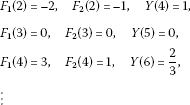Using the inverse transformation rule, we obtain the solution of equation (6) in the form

$y\left(x\right)=-2{x}^{2}+{x}^{4}-\frac{2}{3}{x}^{6}+\cdots .$

In the limit case $k\to \mathrm{\infty }$, we can observe that the series solution obtained by the differential transformation method converges to the series expansion of the closed form solution

$y\left(x\right)=-2ln\left(1+{x}^{2}\right).$

Equation (15) with the coefficient $5/x$ instead of $2/x$ has been solved by Chowdhury and Hashim  using the homotopy perturbation method and Adomian [18, 19] using the Adomian decomposition method. They obtained a closed form solution as well but with the help of many symbolic calculations.

Equation (15) has been also investigated by Yiǧider et al.  using the differential transformation method. They obtained only the series solution (not in the closed form)

$y\left(x\right)=-2{x}^{2}+{x}^{4}-\frac{5}{21}{x}^{6}+\frac{25}{756}{x}^{8}-\cdots ,$

because they came out only from linear approximations of exponential nonlinearities.

Now, we derive the differential transformation of the certain logarithmic nonlinearity of the Lane-Emden type equation which occurs in the stellar structure theory and the thermionic current theory (see [11, 13]).

Theorem 3 If $g\left(y\left(x\right)\right)=y\left(x\right)lny\left(x\right)$, $y\left(0\right)=1$ and $G\left(k\right)$ is the differential transformation of the function $g\left(y\left(x\right)\right)$, then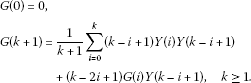(19)

Proof Put

$g\left(y\left(x\right)\right)=y\left(x\right)lny\left(x\right)=\sum _{k=0}^{\mathrm{\infty }}G\left(k\right){x}^{k},\phantom{\rule{1em}{0ex}}y\left(x\right)=\sum _{k=0}^{\mathrm{\infty }}Y\left(k\right){x}^{k}.$
(20)

It is obvious that $G\left(0\right)=0$. Now, we use the following identity:

$y{\left(g\left(y\left(x\right)\right)\right)}^{\prime }=\left(y+g\left(y\left(x\right)\right)\right){y}^{\prime }.$
(21)

If we apply the inverse transformation, we obtain from (20) and Theorem 1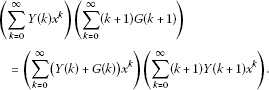Comparing the terms with the same power of ${x}^{k}$, we have

$\sum _{i=0}^{k}\left(i+1\right)G\left(i+1\right)Y\left(k-i\right)=\sum _{i=0}^{k}\left(G\left(i\right)+Y\left(i\right)\right)Y\left(k-i+1\right)\left(k-i+1\right).$
(22)

From (22) we get

$\left(k+1\right)G\left(k+1\right)=\sum _{i=0}^{k}\left(G\left(i\right)+Y\left(i\right)\right)Y\left(k-i+1\right)\left(k-i+1\right)-\sum _{i=0}^{k-1}\left(i+1\right)G\left(i+1\right)Y\left(k-i\right).$
(23)

Replacing $i+1$ by i in the second sum on the right-hand side of identity (9) and considering the fact that $G\left(0\right)=0$, we obtain

$\begin{array}{rcl}G\left(k+1\right)& =& \frac{1}{k+1}\sum _{i=0}^{k}\left(k-i+1\right)Y\left(i\right)Y\left(k-i+1\right)\\ +\left(k-2i+1\right)G\left(i\right)Y\left(k-i+1\right).\end{array}$

The proof is complete. □

Example 3 Consider the following Lane-Emden type equation:

${y}^{″}+\frac{8}{x}{y}^{\prime }+18y+4ylny=0$
(24)

with initial conditions $y\left(0\right)=1$, ${y}^{\prime }\left(0\right)=0$.

Multiplying equation (24) by x and using Theorem 1, we obtain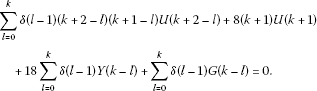(25)

Thus,

$k\left(k+1\right)Y\left(k+1\right)+8\left(k+1\right)Y\left(k+1\right)+18Y\left(k-1\right)+4G\left(k-1\right)=0.$

From here it follows

$Y\left(k+1\right)=-\frac{1}{\left(k+1\right)\left(k+8\right)}\left(18Y\left(k-1\right)+4G\left(k-1\right)\right),\phantom{\rule{1em}{0ex}}k\ge 1.$
(26)

Replacing $k-1$ by k relation (26) gives

$Y\left(k+2\right)=-\frac{1}{\left(k+2\right)\left(k+9\right)}\left(18Y\left(k\right)+4G\left(k\right)\right),\phantom{\rule{1em}{0ex}}k\ge 0$
(27)

and from here we get

$G\left(k\right)=-\frac{1}{4}\left(\left(k+2\right)\left(k+9\right)Y\left(k+2\right)+18Y\left(k\right)\right),\phantom{\rule{1em}{0ex}}k\ge 0.$
(28)

Using (19) for $k\ge 1$, we can write relation (27) in the form

$\begin{array}{rcl}Y\left(k+2\right)& =& -\frac{1}{\left(k+2\right)\left(k+9\right)}\\ ×\left[18Y\left(k\right)+\frac{4}{k}\sum _{i=0}^{k-1}\left(k-i\right)\left(Y\left(i\right)Y\left(k-i\right)+\left(k-2i\right)G\left(i\right)Y\left(k-i\right)\right)\right],\end{array}$

which in view of (28) implies

$\begin{array}{rcl}Y\left(k+2\right)& =& -\frac{1}{\left(k+2\right)\left(k+9\right)}\left[18Y\left(k\right)+\frac{4}{k}\sum _{i=0}^{k-1}\left(k-i\right)\left(Y\left(i\right)Y\left(k-i\right)\\ -\frac{1}{4}\left(Y\left(i+2\right)\left(i+2\right)\left(i+9\right)+18Y\left(i\right)\right)\left(k-2i\right)Y\left(k-i\right)\right)\right].\end{array}$
(29)

From initial conditions and relation (27), we get

$Y\left(0\right)=1,\phantom{\rule{2em}{0ex}}Y\left(1\right)=0,\phantom{\rule{2em}{0ex}}Y\left(2\right)=-\frac{1}{18}\left(18Y\left(0\right)+4G\left(0\right)\right)=-1.$

Then recurrence relation (29) yields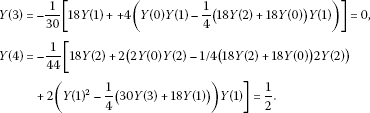Continuing in this way, we obtain

$Y\left(5\right)=0,\phantom{\rule{2em}{0ex}}Y\left(6\right)=-\frac{1}{3!},\phantom{\rule{2em}{0ex}}Y\left(7\right)=0,\phantom{\rule{2em}{0ex}}Y\left(8\right)=\frac{1}{4!},\phantom{\rule{2em}{0ex}}\dots .$

Hence, the solution of the equation has the following form:

$y\left(x\right)=1-{x}^{2}+\frac{{x}^{4}}{2!}-\frac{{x}^{6}}{3!}+\frac{{x}^{8}}{4!}-\cdots .$

If $k\to \mathrm{\infty }$, then the series solution converges to the series expansion of the closed form solution

$y\left(x\right)={e}^{-{x}^{2}}$

as obtained by Chowdhury  by the homotopy perturbation method and by Wazwaz [19, 20] by the Adomian decomposition method. Disadvantage of both mentioned methods is solving many differential equations of the second order or complicated symbolic calculations of so-called Adomian polynomials.

Parand et al.  obtained a series solution of (24) (not in the closed form) using the Hermite functions collocation method.

## Conclusion

The differential transformation method (DTM) is a reliable method applied by providing new theorems to develop exact and approximate solutions of Lane-Emden type equations with exponential and logarithmic nonlinearities. The results obtained with the proposed methods are in good agreement with those obtained by other methods. The main advantage of this method is that it can be applied directly to differential equations without requiring linearization, discretization or perturbation. Another important advantage is that this method is capable of greatly reducing the size of computational work and, as well, the proposed method reduces the solution of a problem to the solution of a system of recurrence algebraic equations. It may be concluded that DTM is very powerful and efficient in finding analytical as well as numerical solutions for wide classes of differential equations.

## References

1. Bert CV, Zeng H: Analysis of axial vibration of compound bars by differential transformation method. J. Sound Vib. 2004, 275: 641–647. 10.1016/j.jsv.2003.06.019

2. Chen CK, Chen SS: Application of the differential transformation method to a non-linear conservative system. Appl. Math. Comput. 2004, 154: 431–441. 10.1016/S0096-3003(03)00723-9

3. Kuo BL: Thermal boundary-layer problems in a semi-infinite flat plate by the differential transformation method. Appl. Math. Comput. 2004, 150: 303–320. 10.1016/S0096-3003(03)00233-9

4. Kuo BL: Heat transfer analysis for the Falkner-Skan wedge flow by the differential transformation method. Int. J. Heat Mass Transf. 2005, 48: 5036–5046. 10.1016/j.ijheatmasstransfer.2003.10.046

5. Malik M, Allali M: Characteristic equations of rectangular plates by differential transformation. J. Sound Vib. 2000, 233(2):359–366. 10.1006/jsvi.2000.2828

6. Davis HT: Introduction to Nonlinear Differential and Integral Equations. Dover, New York; 1962.

7. Zhou JK: Differential Transformation and Its Applications for Electrical Circuits (in Chinese). Huazhong University Press, Wuhan; 1986.

8. Diblík J: A multidimensional singular boundary value problem of the Cauchy-Nicoletti type. Georgian Math. J. 1997, 4(4):303–312. 10.1023/A:1022986311193

9. Diblík J: The singular Cauchy-Nicoletti problem for system of two ordinary differential equations. Math. Bohem. 1992, 117(1):55–67.

10. Diblík J: The asymptotic behavior of solutions of a differential equation partially solved with respect to the derivative (in Russian). Sibirskij matěmatičeskij žurnal 1982, 23(5):80–91.

11. Richardson OU: The Emission of Electricity from Hot Bodies. Longman, Green London; 1921.

12. Chandrasekhar S: Introduction to the Study of Stellar Structure. Dover, New York; 1967.

13. Aslanov A: Determination of convergence intervals of the series solutions of Emden-Fowler equations using polytropes and isothermal spheres. Phys. Lett. A 2008, 372(20):3541–3740. 10.1016/j.physleta.2008.02.024

14. Ramos JI: Series approach to the Lane-Emden equation and comparison with the homotopy perturbation method. Chaos Solitons Fractals 2008, 38(2):400–408. 10.1016/j.chaos.2006.11.018

15. Dehghan M, Shakeri F: Approximate solution of a differential equation arising in astrophysics using the variational iteration method. New Astron. 2008, 13: 53–59. 10.1016/j.newast.2007.06.012

16. Momoniat E, Harley C: Approximate implicit solution of a Lane-Emden equation. New Astron. 2006, 11: 520–526. 10.1016/j.newast.2006.02.004

17. Shawagfeh NT: Nonperturbative approximate solution for Lane-Emden equation. J. Math. Phys. 1993, 34(9):4364–4369. 10.1063/1.530005

18. Wazwaz AM: Adomian decomposition method for a reliable treatment of the Emden-Fowler equation. Appl. Math. Comput. 2005, 166: 638–651. 10.1016/j.amc.2004.06.058

19. Wazwaz AM: Adomian decomposition method for a reliable treatment of the Emden-Fowler equation. Appl. Math. Comput. 2005, 161(2):543–560. 10.1016/j.amc.2003.12.048

20. Wazwaz AM: The modified decomposition method for analytic treatment of differential equations. Appl. Math. Comput. 2006, 173(1):165–176. 10.1016/j.amc.2005.02.048

21. Yang C, Hou J: A numerical method for Lane-Emden equations using hybrid functions and the collocation method. J. Appl. Math. 2012., 2012: Article ID 316534. doi:10.1155/2012/316534

22. Abdel IH, Hassan H: Differential transformation technique for solving higher-order initial value problems. Appl. Math. Comput. 2004, 154: 299–311. 10.1016/S0096-3003(03)00708-2

23. Arikoglu A, Ozkol I: Solution of boundary value problems for integrodifferential equations by using differential transform method. Appl. Math. Comput. 2005, 168: 1145–1158. 10.1016/j.amc.2004.10.009

24. Ertürk VS: Differential transformation method for solving differential equations of Lane-Emden type. Math. Comput. Appl. 2007, 12(3):135–139.

25. Arikoglu A, Özkol I: Inner-outer matching solution of Blasius equation by DTM. Aircr. Eng. 2005, 77: 298–301. 10.1108/00022660510606367

26. Arikoglu A, Özkol I: Solution of difference equations by using differential transform method. Appl. Math. Comput. 2006, 174: 1216–1228. 10.1016/j.amc.2005.06.013

27. Jang MJ, Chen CL, Liy YC: On solving the initial-value problems using the differential transformation method. Appl. Math. Comput. 2000, 115: 145–160. 10.1016/S0096-3003(99)00137-X

28. Gupta VG, Sharma P: Solving singular initial value problems of Emden-Fowler and Lane-Emden type. Int. J. Appl. Math. Comput. Sci. 2009, 1(4):170–177.

29. Rafig A, Hussain S, Ahmed M: General homotopy method for Lane-Emden type differential equations. Int. J. Appl. Math. Mech. 2009, 5(3):75–83.

30. Rafig A, Hussain S, Ahmed M: A general approach to specific second order ordinary differential equations using homotopy perturbation method. Phys. Lett. A 2008, 327(30):4973–4976.

31. Parand K, Dehghanb M, Rezaeia AR, Ghaderia SM: An approximation algorithm for the solution of the nonlinear Lane-Emden type equations arising in astrophysics using Hermite functions collocation method. Comput. Phys. Commun. 2010, 181: 1096–1108. 10.1016/j.cpc.2010.02.018

32. Yildirim A, Özis T: Solutions of Singular IVPs of Lane-Emden type by the variational iteration method. Nonlinear Anal., Theory Methods Appl. 2009, 70: 2480–2484. 10.1016/j.na.2008.03.012

33. Chowdhury MSH, Hashim I: Solutions of Emden-Fowler equations by homotopy perturbation method. Nonlinear Anal., Real World Appl. 2009, 10: 104–115. 10.1016/j.nonrwa.2007.08.017

34. Yiǧider M, Tabatabaei K, Celik E: The numerical method for solving differential equations of Lane-Emden type by Padé approximation. Discrete Dyn. Nat. Soc. 2011., 2011: Article ID 479396. doi:10.1155/2011/479396

## Acknowledgements

The second author is supported by the Grant FEKT-S-11-2-921 of the Faculty of Electrical Engineering and Communication, Brno University of Technology. The third author is supported by the Grant P201/11/0768 of the Czech Grant Agency (Prague).

## Author information

Authors

### Corresponding author

Correspondence to Zdeněk Šmarda.

### Competing interests

The authors declare that they have no competing interests.

### Authors’ contributions

YK proved the theorem which gives differential transformation formula for exponential nonlinearity. ZS illustrated obtained results with examples. ZS proved the theorem which gives differential transformation formula for logarithmic nonlinearity and wrote the first and corrected version of the paper. All authors read and approved the final manuscript.

## Rights and permissions

Open Access This article is distributed under the terms of the Creative Commons Attribution 2.0 International License ( https://creativecommons.org/licenses/by/2.0 ), which permits unrestricted use, distribution, and reproduction in any medium, provided the original work is properly cited.

Reprints and Permissions

Khan, Y., Svoboda, Z. & Šmarda, Z. Solving certain classes of Lane-Emden type equations using the differential transformation method. Adv Differ Equ 2012, 174 (2012). https://doi.org/10.1186/1687-1847-2012-174

• Accepted:

• Published:

• DOI: https://doi.org/10.1186/1687-1847-2012-174

### Keywords

• Series Solution
• Symbolic Calculation
• Homotopy Perturbation Method
• Variation Iteration Method
• Adomian Decomposition Method